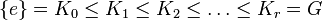# Finite nilpotent implies every normal subgroup is part of a chief series

Suppose$G$ is a Finite nilpotent group (?) and$H$ is a normal subgroup of$G$. Then, there exists a Chief series (?) for$G$ that has$H$ as one of its members, i.e., there is a series:$\{ e \} = K_0 \le K_1 \le K_2 \le \dots \le K_r = G$
such that each$K_i$ is a normal subgroup of$G$ and there exists some value of$i$ for which$K_i = H$.